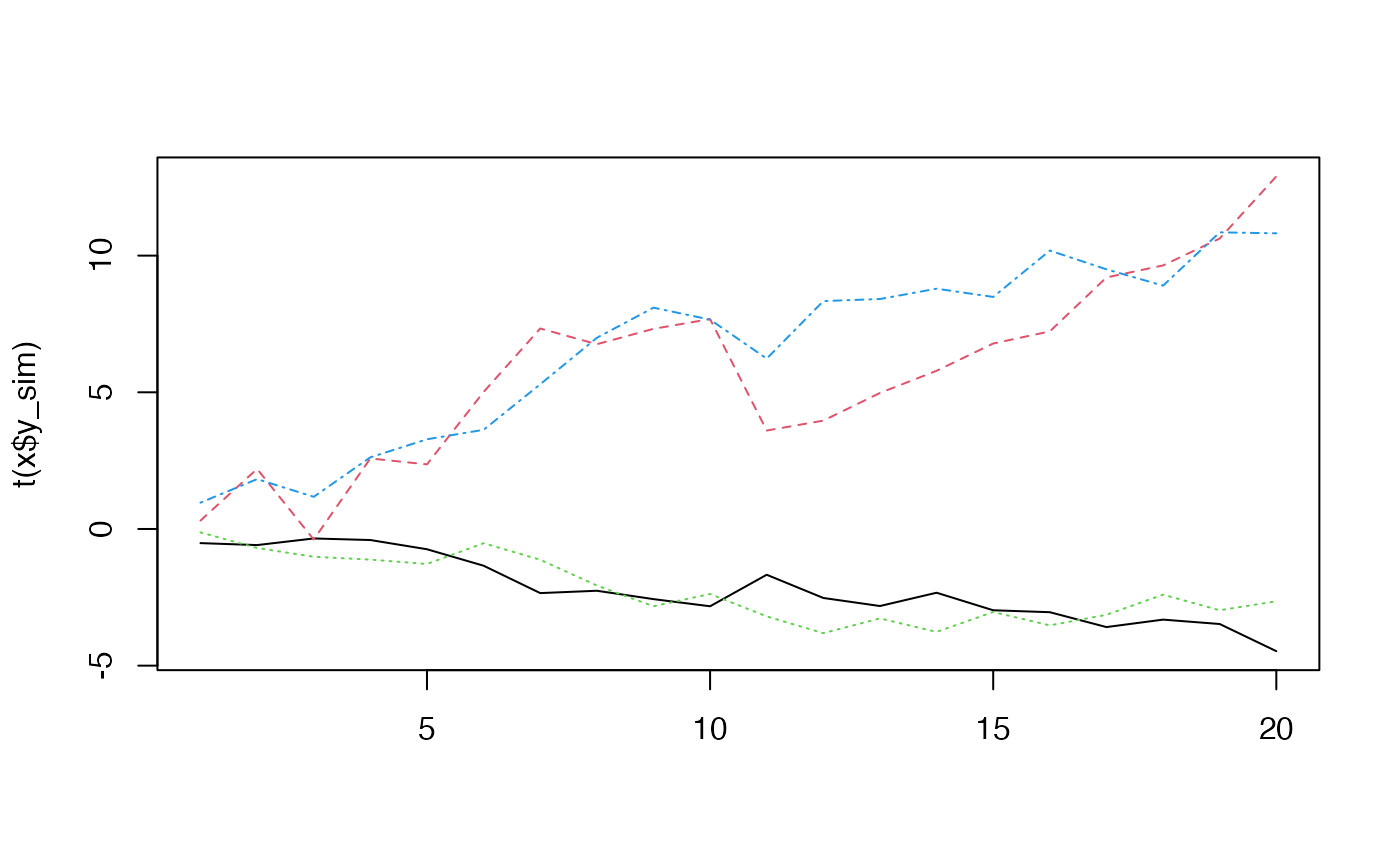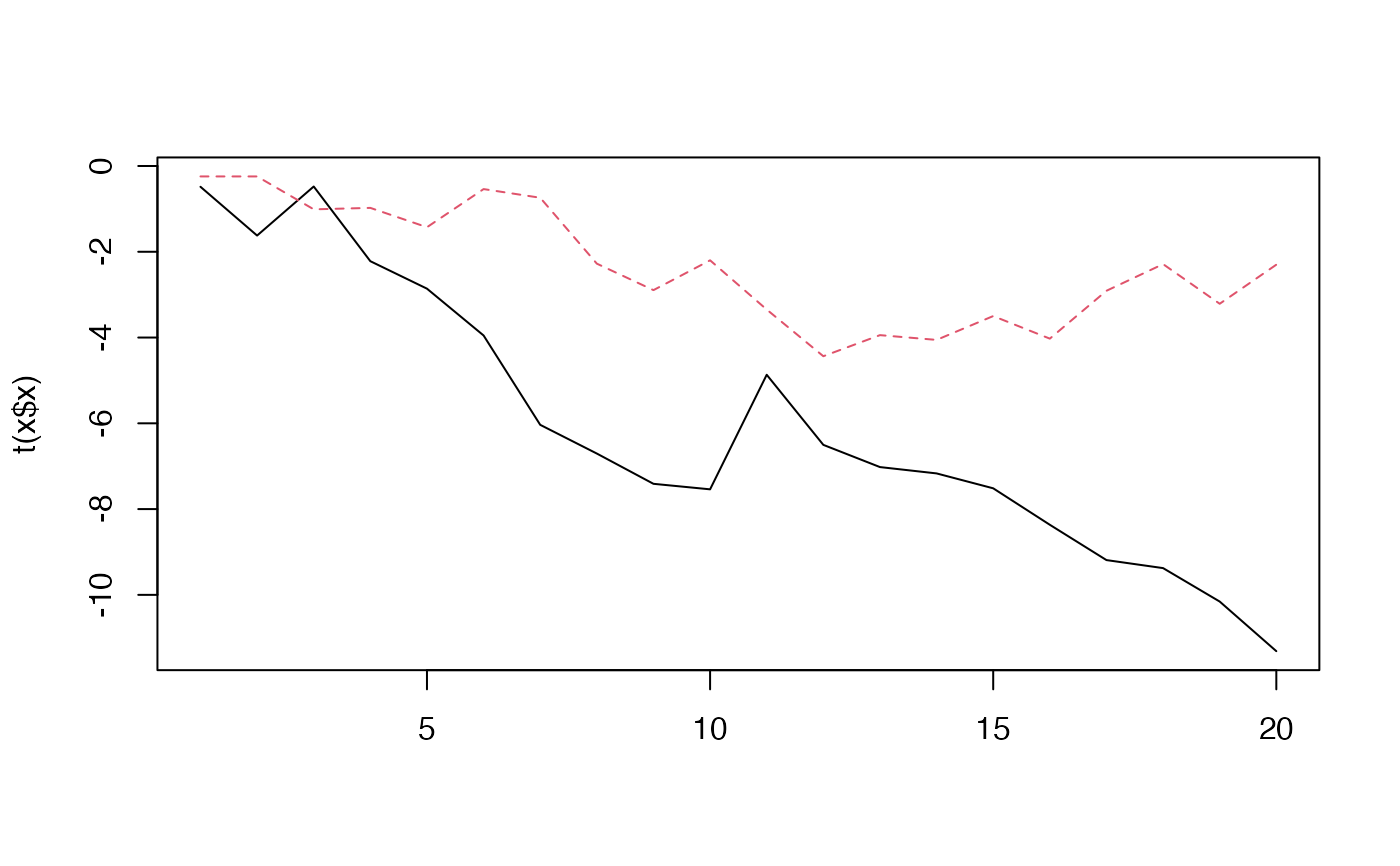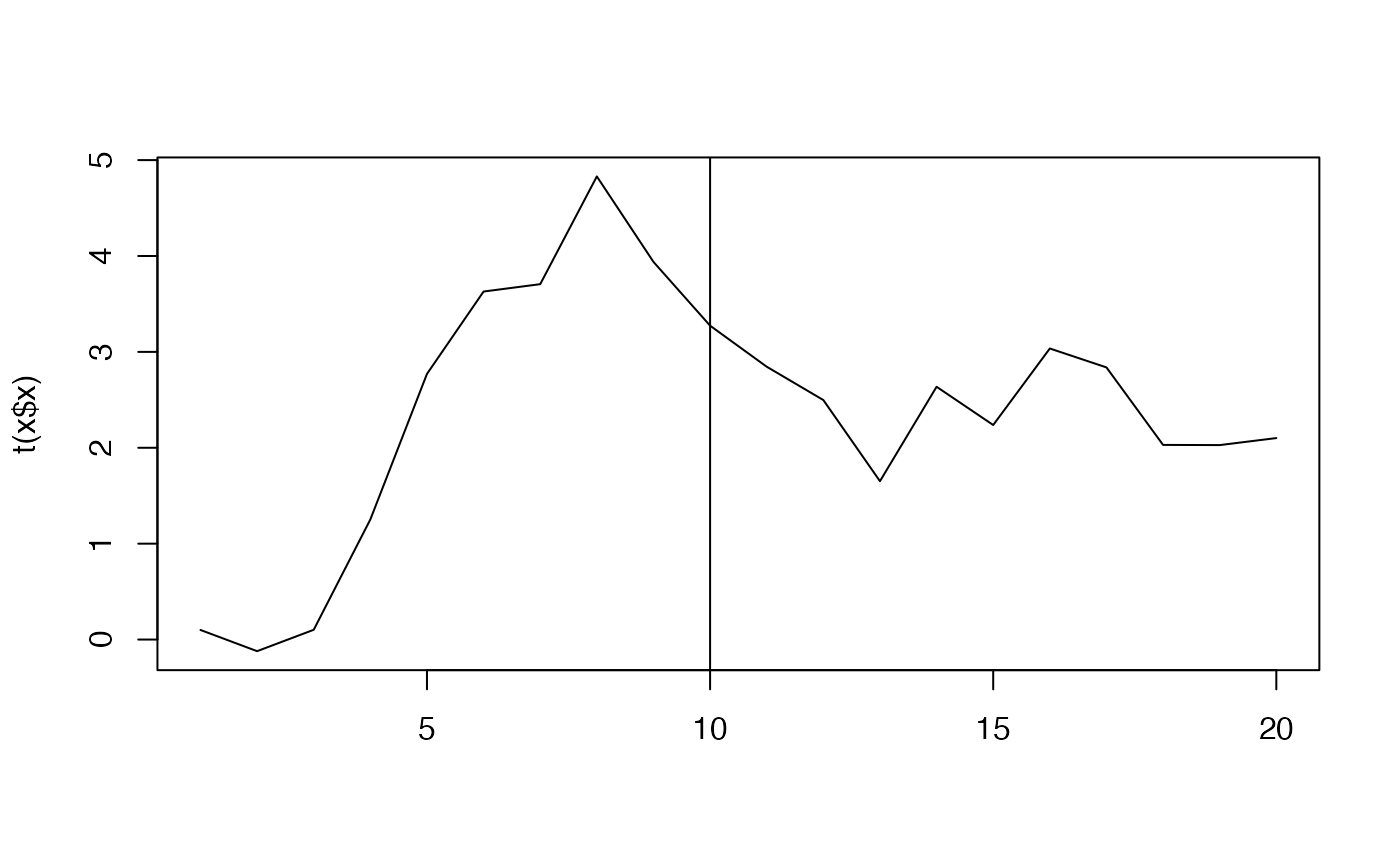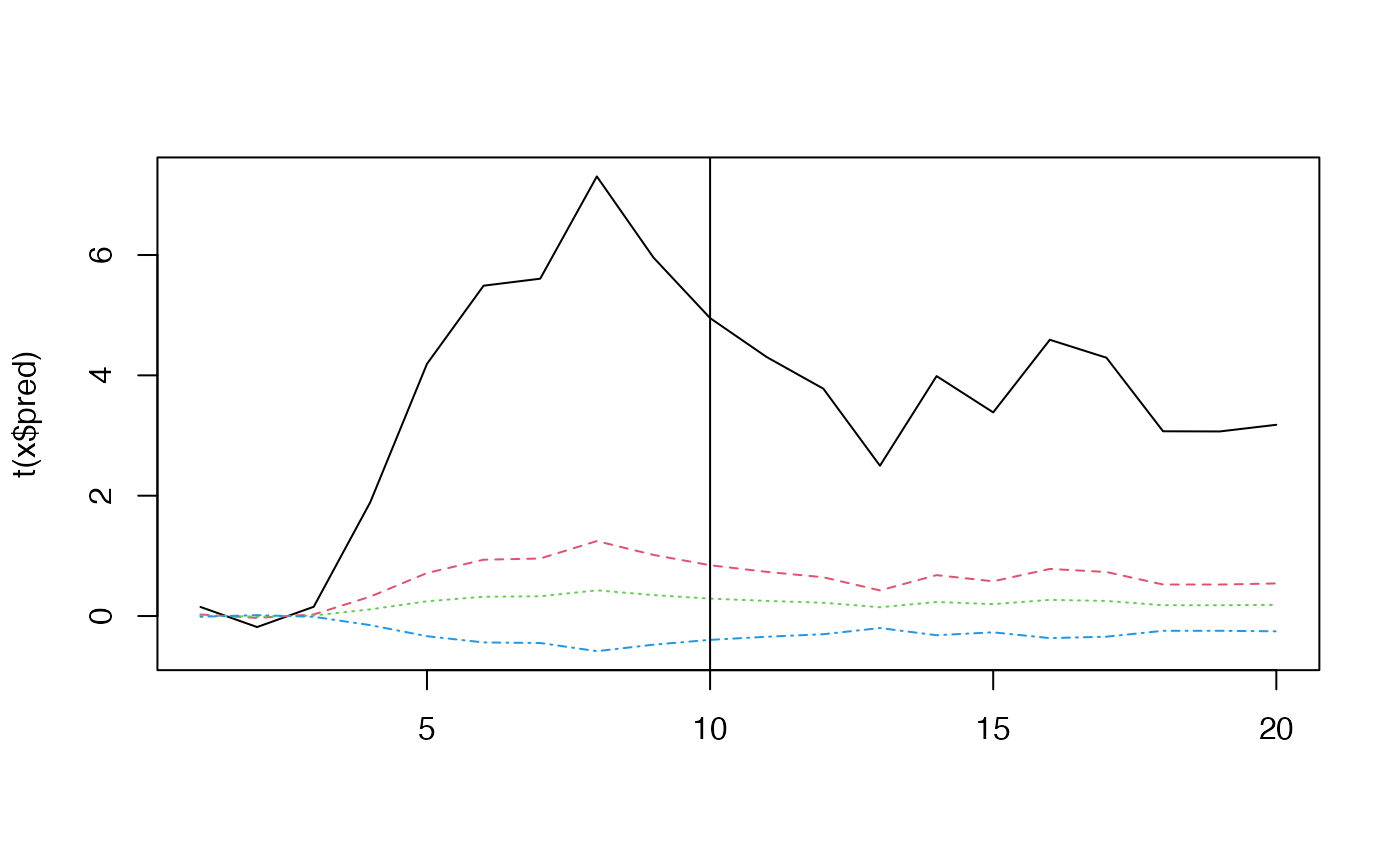Simulate from a DFA

sim_dfa(
num_trends = 1,
num_years = 20,
num_ts = 4,
0, 1)),
sigma = rlnorm(1, meanlog = log(0.2), 0.1),
varIndx = rep(1, num_ts),
extreme_value = NULL,
extreme_loc = NULL,
nu_fixed = 100,
user_supplied_deviations = NULL
)

## Arguments

num_trends The number of trends. The number of years. The number of timeseries. A loadings matrix. The number of rows should match the number of timeseries and the number of columns should match the number of trends. Note that this loadings matrix will be internally manipulated by setting some elements to 0 and constraining some elements to 1 so that the model can be fitted. See fit_dfa(). See the outfit element Z in the returned list is to see the manipulated loadings matrix. If not specified, a random matrix ~ N(0, 1) is used. A vector of standard deviations on the observation error. Should be of the same length as the number of trends. If not specified, random numbers are used rlnorm(1, meanlog = log(0.2), 0.1). Indices of unique observation variances. Defaults to c(1, 1, 1, 1). Unique observation error variances would be specified as c(1, 2, 3, 4) in the case of 4 time series. Value added to the random walk in the extreme time step. Defaults to not included. Location of single extreme event in the process. The same for all processes, and defaults to round(n_t/2) where n_t is the time series length Nu is the degrees of freedom parameter for the t-distribution, defaults to 100, which is effectively normal. An optional matrix of deviations for the trend random walks. Columns are for trends and rows are for each time step.

## Value

A list with the following elements: y_sim is the simulated data, pred is the true underlying data without observation error added, x is the underlying trends, Z is the manipulated loadings matrix that is fed to the model.

## Examples

x <- sim_dfa(num_trends = 2)
names(x)
#>  "y_sim" "pred"  "x"     "Z"     "sigma"matplot(t(x$y_sim), type = "l")matplot(t(x$x), type = "l")set.seed(42)
x <- sim_dfa(extreme_value = -4, extreme_loc = 10)
matplot(t(x$x), type = "l") abline(v = 10)matplot(t(x$pred), type = "l")
abline(v = 10)set.seed(42)
x <- sim_dfa()
matplot(t(x$x), type = "l") abline(v = 10)matplot(t(x$pred), type = "l")
abline(v = 10)